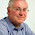# Artificial Intelligence Questions and Answers Set 3

## Artificial Intelligence

### Questions 21 to 30

 21 Which is not a desirable property of a logical rule-based system? (a)  Locality                                            (b)  Attachment                     (c)  Detachment (d)  Truth-Functionality                            (e)  Global attribute. 22 How is Fuzzy Logic different from conventional control methods? (a)  IF and THEN Approach                      (b)  FOR Approach (c)  WHILE Approach                                                            (d)  DO Approach (e)  Else If approach. 23 In an Unsupervised learning (a)   Specific output values are given (b)   Specific output values are not given (c)   No specific Inputs are given (d)   Both inputs and outputs are given (e)   Neither inputs nor outputs are given. 24 Inductive learning involves finding a (a)  Consistent Hypothesis                      (b)  Inconsistent Hypothesis (c)  Regular Hypothesis                           (d)  Irregular Hypothesis (e)  Estimated Hypothesis. 25 Computational learning theory analyzes the sample complexity and computational complexity of (a)  UnSupervised Learning                      (b)  Inductive learning (c)  Forced based learning                       (d)  Weak learning (e)  Knowledge based learning. 26 If a hypothesis says it should be positive, but in fact it is negative, we call it (a)  A consistent hypothesis                    (b)  A false negative hypothesis (c)  A false positive hypothesis                (d)  A specialized hypothesis (e)  A true positive hypothesis. 27 Neural Networks are complex -----------------------with many parameters. (a)  Linear Functions                               (b)  Nonlinear Functions (c)  Discrete Functions                            (d)  Exponential Functions (e)  Power Functions. 28 A perceptron is a --------------------------------. (a)  Feed-forward neural network              (b)  Back-propagation alogorithm (c)  Back-tracking algorithm                     (d)  Feed Forward-backward algorithm (e)  Optimal algorithm with Dynamic programming. 29 Which is true? (a)   Not all formal languages are context-free (b)   All formal languages are Context free (c)   All formal languages are like natural language (d)   Natural languages are context-oriented free (e)   Natural language is formal. 30 Which is not true? (a)   The union and concatenation of two context-free languages is context-free (b)   The reverse of a context-free language is context-free, but the complement need not be (c)   Every regular language is context-free because it can be described by a regular grammar (d)   The intersection of a context-free language and a regular language is always context-free (e)   The intersection two context-free languages is context-free.

#### Answers

 21 Answer : (b) Reason : Locality: In logical systems, whenever we have a rule of the form A => B, we can conclude B, given evidence A, without worrying about any other rules. Detachment: Once a logical proof is found for a proposition B, the proposition can be used regardless of how it was derived .That is, it can be detachment from its justification. Truth-functionality: In logic, the truth of complex sentences can be computed from the truth of the components. But there are no Attachment properties lies in a Rule-based system. Global attribute defines a particular problem space as user specific and changes according to user’s plan to problem. 22 Answer : (a) Reason : FL incorporates a simple, rule-based IF X AND Y THEN Z approach to a solving control problem rather than attempting to model a system mathematically. The FL model is empirically-based, relying on an operator's experience rather than their technical understanding of the system. For example, rather than dealing with temperature control in terms such as "SP =500F", "T <1000F", or "210C

<< Prev  1 ...  2 ...  3 ...  4 ...  5 ...  6 ...  Next  >>

#### 4 comments :

1.A perceptron is a Feed-forward neural network with no hidden units that can be represent only linear separable functions. If the data are linearly separable, a simple weight updated rule can be used to fit the data exactly.speech recognition software

2.If you want your ex-girlfriend or ex-boyfriend to come crawling back to you on their knees (even if they're dating somebody else now) you have to watch this video
right away...

(VIDEO) Why your ex will NEVER come back...

3.4.This information is impressive; I am inspired how continuously you describe this topic. After reading your post, thanks for taking the time to discuss this, I feel happy about it and I love learning more about this topic snowflake data warehouse